# Math Manipulatives: Calculators, Calculator Apps for Mobile Devices, and Related ResourcesMath Manipulatives contains three pages of resources:## Calculator Opening StatementsThere are many kinds of online and hand-held calculators.  Some are dedicated to specific professional fields; others have features beyond the capabilities of learners to use those, or features that learners might not be able to use on state standardized testing.  Some displays of online calculator input or output are easier to work with than others.  Therefore, educators should help learners choose those appropriate for the level of math they are learning, thus avoiding the potential problem of "teaching one way, but testing another way."

Consider the following.  A graphing calculator is an essential tool for learners in mathematics courses such as algebra, trigonometry, advanced math, and calculus.  The visual display becomes a powerful tool for teaching and learning to show the link among conceptual, procedural, analytic, and investigative dimensions of learning mathematics.  When it comes to using a graphing calculator (or some scientific calculators with certain non-graphing features) on state standardized testing, there may be restrictions on use that educators should be aware of depending on grade level of the learner taking the exam.

## Calculator Tutorials, Activities, and Software Enhancements

Manufacturers and technology organizations offer tutorials and manuals for elementary, financial, graphing, and scientific calculators.  Examples follow.

Casio Education training for Casio calculators.

SimCalc MathWorlds software for TI-graphing calculators, computer, and TI-Navigator was developed by the University of Massachusetts-Dartmouth's James J. Kaput Center for Research and Innovation in STEM Education.  Animations, real life examples, narrative stories, and more are used to explain math concepts.

Sharp scientific calculators educational support

Texas Instruments Classroom Activities are free, standards-aligned, and designed for use with Texas Instrument graphing calculators.  You'll find activities for the TI-84 and MathNspire in various subjects such as middle grades math,  algebra 1, algebra 2, geometry, precalculus, calculus, statistics, and math modeling.  There are sections called TI Code and Building Concepts in Mathematics, activities aligned to TEKS, CCSS, and more.  Tutorials are available for TI calculators.  Note: MathBits.com includes a series of tutorials on Finding Your Way Around the TI-84 Plus Family of Calculators.  An extensive collection of tutorials are presented within subjects.

## Online Calculators and PDA ResourcesNote: Several of the following resources are free for online use or download.

Accessible Calculators --this list provides information on the types of accessible calculators and potential sources.  It was developed at the Georgia Tech Research Corporation Center for Assistive Technology and Environmental Access.

Analyze Math Online Maths Calculators and Solvers for multiple topics in math.

Calc 3D includes downloadable mathematical graph and charting software for geometry and statistics.  The calculator can do statistics, best fits, function plotting, polar and parametric plotting, integration. It handles vectors, matrices, complex numbers, quaternions, coordinates (Cartesian, polar, spherical, cylindrical), regular polygons, and intersections.  Find distances, volume, area, and more.  The software handles several languages, too.  Online calculators are also available.

CalculateWhat.com features a variety of free online calculators for just about anything, with a large emphasis on math calculators for algebra, geometry, statistics, and general math.  Each page also offers formula information and a brief background on each particular subject.  New calculators are added regularly.

Calculator.net has an extensive collection of calculators in categories for math, financial, fitness & health, and other.  Math calculators are arranged for basic math, statistics, and geometry.  Explanations are included.

Calculatores.com features an extensive collection of calculators for math on multiple topics, and includes sections for derivatives, surface area, matrices, percentages, and much more.

Calculators.org contains a collection of free online calculators on the Web organized by theme: savings, loans, debt, budget, automotive, credit cards, investing, mortgage, math, retirement, business, and health.  Math calculators from basic math to calculus are arranged by subject, topic, and level of difficulty.

Calculator Soup contains free online calculators, many of which also provide work and explanations to help understand the calculations.  They are grouped by categories: mathematics, financial (e.g., interest), and other (e.g., conversions).  Math includes calculators on fractions, general math, algebra, discrete math, geometry, plane and solid geometry, statistics, trigonometry, and word problems.

Calculus: The Online Integral Calculator from Wolfram Alpha.  Enter any expression and the integration is performed for you.  Also read about the history and applications of integration.

ClassPad.net is a "FREE all-in-one, web based calculation tool that includes Calculation, Graphing, Geometry and Statistics, free to teachers and students" (Home page description).  Video tutorials are available.  ClassPad.net provides features based on technologies of CASIO CAS graphic calculators.

DadsWorksheets.com has thousands of math worksheets on multiple topics and a series of online calculators (e.g., prime factors, factors, degrees to radians with visual on a circle, slope and related linear equation with visual on a graph, percentage, fractions, whole number multiplication, long division, and more).

Derivative Calculator is free and finds derivatives of functions.  "The Derivative Calculator supports computing first, second, …, fifth derivatives as well as differentiating functions with many variables (partial derivatives), implicit differentiation and calculating roots/zeros. You can also check your answers! Interactive graphs/plots help visualize and better understand the functions" (About section).

HOT!: Desmos Graphing Calculator is free from Desmos.com and compatible with any computer or tablet.  Desmos also includes tutorials for what you can do with Desmos and classroom activities.  Desmos also has a tool for geometry, and has added four-function, matrix, and scientific calculators.  Note: Desmos is included on over 30 state assessments.  Desmos developed a suite of "Test Mode" apps to "help students and teachers prepare for these assessments—and to support delivery of lower-stakes tests and quizzes in classrooms."  This is a winner for math educators!  Users who desire an additional tutorial might read Desmos: A Definitive Guide on Graphing and Computing from Math Vault.

EasyCalculation has a series of free online calculators set up as they relate to specific math concepts for number, algebra, statistics, geometry, trigonometry, calculus, and more.  There are also some tutorials on the math concepts related to those calculations.

EasyUnitConverter includes calculator and metric converters in multiple categories.eMathHelp Calculators are available for multiple subjects, such as pre-algebra, algebra, geometry, calculus, statistics & probability, differential equations, and more.  Each subject has specific calculators devoted to topics studied within each.  Step-by-step notes related to the solution of each calculation are included.  The site is free.

Geogebra Graphing Calculator

Good Calculators has over 200 free online calculators grouped into 24 categories for easy selection.  These are useful in school settings, business, and for math in daily life.  Insight into how calculations are derived is provided where possible.  Calculators are by John Sanders and Andrew Stacy.  For example, see the categories: real-function calculators, volume calculators, 2D and 3D shape calculators, statistics calculators.

GraphCalc is a Windows 2D/3D graphing calculator.  Download it for free.  The developers call it "an all-in-one solution to everything from everyday arithmetic to statistical analysis, from betas to Booleans, from cubes to calculus, from decimals to derivatives. GraphCalc combines all the features of a professional mathematics package with the simplicity of an easy to learn windows interface. It provides user-friendly help and tutorials to guide you through the easy and fun process of mastering GraphCalc."  A Linux version is also available.

Had2Know contains online calculators in eight categories: math, business and finance, home and garden remodeling, science, technology, health, automotive, and games and sports.  The math calculators are in categories of algebra and analysis, basic student math, geometry, numbers and number theory, and statistics.  There is also a scientific calculator.  Explanatory articles are included.

InstaCalc is a free online calculator that can interpret natural language expressions and equations.  It also includes instructions for getting started. Results are displayed instantly, and can be shared.  Perform basic math, convert units of measure and currency, use variables and rows, create charts similar to what you'd do with spreadsheets, work with trigonometry, logarithms, programming tools, and more.  You can add notes and embed your calculations in your own web pages, too.  It's amazing.

Integral Calculator is free and finds integrals and antiderivatives of functions.  It shows you the full step by step integration.  "All common integration techniques and even special functions are supported. The Integral Calculator supports definite and indefinite integrals (antiderivatives) as well as integrating functions with many variables. You can also check your answers! Interactive graphs/plots help visualize and better understand the functions" (About section).

HOT!: Math Portal Online Calculators and Solvers by mathematician Miloš Petrović contains multiple math calculators and solvers grouped into 17 categories.  Use this site for problems including polynomial equations, rational expressions, systems of equations, vectors, matrices, complex numbers, plane and analytic geometry, statistics, trigonometry, calculus (limits, derivatives, integrals), sequences, series, financial, numbers, and more.  Per the description, "These calculators can help you with basic and advanced problems in high school and college math.  All of them are capable of performing exact computations.  Also, almost every of them will generate a step by step explanation."  The step by step explanations are exceptionally valuable for learners.  There are three other math sections on the site: formulas, tests and lessons.  Math lessons relate to algebra, calculus, analytic geometry, and linear algebra, and also include solved problems and examples for students to solve.

Math is Fun: Matrix Calculator enables the user to work with matrices up to 10 x 10.  Operations include addition, subtraction, multiplication, finding a determinant, an inverse, squaring a matrix, and more.

HOT: Mathway is a free online problem solver for basic math, pre-algebra, algebra, trigonometry, precalculus, calculus, statistics, finite math, linear algebra, and graphing.

MatrixCalc.org: Matrix Calculator helps the user to find the determinant of a matrix, the rank, raise a matrix to a power, find sum and multiplication of matrices, calculate the inverse matrix.  Enter the matrix elements and click a button.  You can also solve systems of equations using matrices.Matrix Calculator developed by PJ in Belgium enables the user to work with matrices up to 10 x 10.  The calculator will add, subtract, multiply, divide, transpose, find an inverse, find a determinant, and raise to a power.  The beauty of this calculator is that each answer comes with a step-by-step, easy to understand, solution.  Users can show instructions for each operation, which are clearly written and help to understand the math.

HOT!: Meta-Calculator is a free online calculator, which the developer (Vernon Morris, a math and computer science educator) indicated "can indeed do most of the important things that the TI's can do" (personal communication, July 27, 2013).  It's a graphing calculator, scientific calculator, matrix calculator, and statistics calculator.  A blog is included at the site.  Key features:

• Graph up to seven equations, including equations like circles and hyperbolas; find intersections.  Work with common functions such as square root, trig, logarithmic, and inverse functions.  Choose radian or degree mode, set domain and range, produce table of values, plot points.  You can also graph polar equations.  Save the image of your graph to your computer.
• The scientific calculator performs all the basic functions you'd expect and there are advanced features.  You can find the least common multiple, permutations, and combinations.  There's a linear equations solver that lets you input up to six equations with either two or three variables and the solver will calculate the solutions.  Store calculations using the memory button.
• Add, subtract, multiply, and transpose matrices.  Calculate the determinant or inverse of a matrix.
• Statistical calculations include finding quartiles, mean, median, mode, standard deviation, correlation coefficients, and almost any kind of regression (e.g., linear, quadratic, cubic, exponential, power, log, natural log).  Regressions can be graphed.  Also perform T-tests (paired and unpaired, 1 or 2-tailed).

HOT!: Microsoft Math Solver is free and available online and as an app for iOS and Android.  You can get step-by-step solutions to math problems for multiple subjects from basic math to pre-algebra, algebra, trigonometry, calculus, matrices, statistics.  You can graph equations, get practice problems, associated videos for further explanations.  It works in several languages.

HOT!: Omnicalculator.com has over 3000 online calculators designed "to make your life easier."  They are in multiple categories, such as business, construction, conversion, finance, fitness, health, math, physics, and statistics.  Each has a specific purpose.  Several come with additional explanations on the concept.

Online Metric and US Standard Conversions Calculator by Science Made Simple, Inc. contains online measurement conversions for metric and US customary units, such as area, volume, speed, temperature, time, weight, currency, length/height, power, and stress.

Online Statistics Calculators:  There are five statistics calculators Alcula.com: measures of central tendency and dispersion, box and whisker plots, linear regression, correlation coefficients, and scatter-plots.  Other online calculators for math are also available.

Pigly is a free online personal finance guide that also offers over 100 financial calculators.

Statistics Calculators at DanielSoper.com are free for the research community with 29 categories available.

HOT!: Symbolab Equation Calculator "allows users to search and solve equations, formulas and expressions using mathematical symbols and scientific notations as well as text. Symbolab solves math problems." It includes step-by-step calculators for pre-algebra, algebra, functions, geometry, statistics, trigonometry, matrices and vectors, pre-calculus, calculus, finance and conversion topics covered in middle school math through college.  It includes graphing and a math practice section with problems by subject and topic.  Note: This can be a valuable tool.  For example, algebra includes solving equations (e.g., linear, quadratic, absolute value, log, radical, etc.), inequalities, systems of equations, line equations, basic operations.  For trig, you can prove identities and solve trig equations in degrees or radians.  For calculus, work with limits, integrals, derivatives (including implicit), derivative applications (including tangents and normals to curves), and series.  Examples with step by step solutions are provided.  Results of queries can be saved, including notes, and then shared with others.

Talking Calculator is an onscreen full-function talking calculator that can be used with or without a screen reader.  "Every button and edit area talks. It is easy to use with large keys and contrasting colors. It has three display areas, so when the user adds a series of numbers, the total is always displayed, even as you are entering a new number, while always displaying any numbers in memory." Further, "it displays your entries and results as you work. It actually displays the equation so that you can easily see or hear your last process. When students are required to show their work, they can simply cut and paste the steps into a document."  Perform simple and basic operations.  A free download is available.

The Calculator Site by Alastair Hazell contains multiple free online calculators and converters.  There are four sections: financial calculators, unit conversions, health and math calculators, and miscellaneous calculators.  HOT!: Math educators will appreciate the fractions calculators.  The first will add, subtract, multiply or divide fractions, including mixed numbers, and display the answer in fraction and number form.  The second will simplify fractions, using the greatest common factor, and show the result as a fraction and number.  Both also show the explanations on how answers were derived and if the number form is a repeating decimal, a bar is shown over digits that repeat--a very unique feature to such calculators.  The third converts a decimal to a fraction.  A unique feature of this latter is that it also accommodates converting repeating decimals to fractions by also entering the number of decimal places that repeat.  The output shows the repeating decimal with a bar over the digits that repeat and the equivalent fraction.

Unit Conversion from PDFConverter.com is a free series of unit converters with multiple options for converting mass, time zones, area, angle, length, volume, pressure, temperature, and data storage.  Quick and easy to use.Visual Fractions by Richard Rand contains an extensive collection of online fraction and math calculators, including percentages, unit conversions, and more.  The site also includes games, lessons, a visual approach to learning fractions, fraction worksheets (practice with line and circle models), and a fraction designer page where you can create your own examples.

Zona Land's graphics calculator, EZ Graph, enables you to graph almost any polynomial, rational, exponential, logarithmic, or trigonometric function.HOT News!  Google Search can now be used to get help with math, science, and visual learning.  This new search capability, powered by AI, provides step-by-step explanations and solutions for math problems, even trigonometry and calculus, and science word problems.  You can get 3D interactive diagrams to explore STEM concepts visually.  Just type your equation or integral into the Search bar, or enter the phrase "math solver."  The feature is also available on Google Lens where you can also take a picture of your problem.  Read Google's news reported on October 30, 2023: More help with math and science problems in Search.

## Calculator Apps for Mobile DevicesCalcMadeEasy Pro is designed for iPhone and iPad and available for \$1.99.  It's designed to enable "financial calculations/decision making, scientific/mathematical calculations, unit conversions and automatic notes." CalcMadeEasy free scientific calculator with auto notes for Mac is also available.Learners with dyscalculia might benefit from a special talking calculator called the Dyscalculator.  It is designed to assist with arithmetic by offering four different representations of quantities: as digits, as words, graphically, and spoken aloud.  It can also round numbers.  This free app is designed for iPad, iPhone.  Also see Dyscalculator for Android.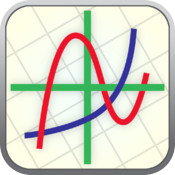Ezy Graph by KGiants is for iPad and iPhone.  This free app with in-app purchases for high school learners and above supports most graphing functions and comes with advanced graph analysis (e.g., domain, zeros, max/min/inflection points of graphs), multiple graphs on a set of axes, customized graphs with color and line style, and trace mode for coordinates of points.  You can share, save, and print graphs.Fraction Calculator Plus by Digitalchemy, LLC is a free app with in-app purchases compatible for use with iPhone, iPod touch, and iPad.  Anyone working with fractions to add, subtract, multiply, or divide can benefit.  Output is displayed in lowest fraction terms and in decimal format.Kalkulilo (scientific calculator) by Alexander Clauss is a calculator app for iPhone and iPad for \$1.99 with in-app purchases.  It comes with several modes.  The scientific mode includes the usual functions and operations such as basic arithmetic, powers, roots, logarithms, trigonometry, and so on.  A bit/integer mode offers logic operations and calculations in 8, 16, 32, or 64 bits.  The statistics mode enables calculations of mean, median, mode, quartile values, variance, standard deviation, range, and so on.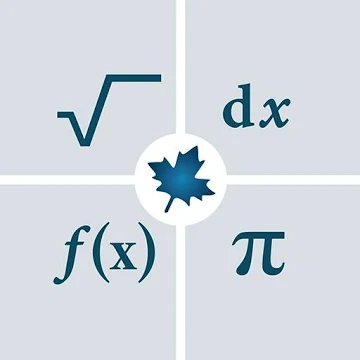Maple Calculator is a free app available on Google Play and in the App Store.  Per its description, "It is a scientific calculator, a differentiation and integration calculator, a matrix calculator, a polynomial manipulation tool, an equation solver, a graphing tool, and so much more!  This versatile app makes it incredibly easy to enter, solve, and visualize mathematical problems from algebra, precalculus, calculus, linear algebra, and differential equations, for free! You can even enter problems using your camera, so you can check your homework with a click of a button."Mathpix: Snip is a scanner app for math and science.  It "digitizes handwritten or printed text, and copies outputs to the clipboard that can be pasted into LaTeX editors like Overleaf, Markdown editors like Typora, Microsoft Word, and more."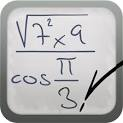MyScript Calculator by MyScript is for iPad and iPhone.  Price: \$2.99.  Enter your expression using symbols and numbers in your own handwriting and this app converts it to digital text and performs the calculation.  The calculator supports basic operations, powers, roots, exponentials, logarithms (ln and log) trig, percents, absolute value, factorials, using brackets, and includes constants (pi, e, phi).Photomath, developed by Microblink, reads and solves math problems by using the camera on your mobile device (iPhone, iPad, or Android) to capture the problem.  It recognizes handwriting, provides step-by-step instructions and explanations, allows for keyboard problem entry.  Per its description: "Photomath supports arithmetics, integers, fractions, decimal numbers, roots, algebraic expressions, linear equations and inequalities, quadratic equations and inequalities, absolute equations and inequalities, systems of equations, logarithms, trigonometry, exponential and logarithmic functions, derivatives and integrals."  The app is free, but does offer in-app purchases.  Graphing has been added in version 4.0.1.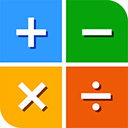Pomegranate Software Calculators and apps for mobile devices include those for iPad, iPhone, Android, and Mac. For example, see the calculator Solve for \$1.99.  It also supports graphing 2D and 3D functions using the x, y and θ variables.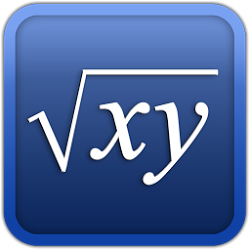Symbolic Calculator by Voxeloid Kft. is for iPhone or iPad, and available for free.  "Symbolic Calculator is capable of helping with any kind of math problems ranging from basic arithmetics to university-level advanced math. It offers all the advantages of an advanced graphing/scientific calculator mixed with the convenience of modern mobile apps."Talking Scientific Calculator by Adam Croser is designed for iPad and iPhone (\$4.99).  It "has large colorful buttons, optional high contrast, full VoiceOver support, and unique to this calculator; the option to use speech for answers, buttons names and formulas."  Supports entry and display of Braille on supported devices.  The accessibility features on this app also make it highly suitable for use by learners with disabilities.TI-Nspire and TI-Nspire CAS (Computer Algebra System) graphing calculator apps for iPad are from Texas Instruments. These apps enable "comprehensive graphing, data entry and analysis, statistical modeling and calculating functionality" (Description section).

### Here's a bit of math history for you!

#### From Slates, to Slide Rules and Software!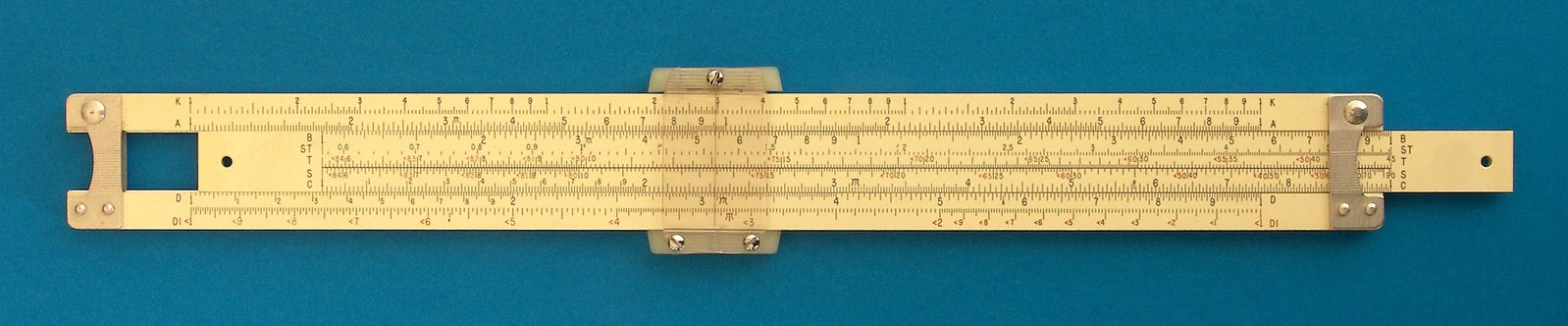Does anyone remember the slide rule?  "Throughout American history, teachers and parents have used objects--from colonial--era slates to modern electronic calculators--to help students master abstract mathematical concepts," according to the National Museum of American History, the Smithsonian Institution (2002), which has posted a highly informative display called Slates, Slide Rules, and Software: Teaching Math in America.  Read developments in math education and teaching with manipulatives from the Early Republic, to the Cold War, and Information Age.  Additional resources are provided.

#### Did you know?"The slide rule has a long and distinguished ancestry … from William Oughtred in 1622 to the Apollo missions to the moon ... a span of three and a half centuries … it was used to perform design calculations for virtually all the major structures built on this earth during that long period of our history … an amazing legacy for something so mechanically simple" (The Oughtred Society, 2021).  Read more about the Slide Rule History and other calculating instruments at the Oughtred Society.

The ways that people have been computing dates back thousands of years.  The tools have changed of course.  See the Chronological Calculator Timeline, which dates clay tablets being used for commercial transactions by Sumerians around 4000-12000 BC.  See key events to the present time.

Calculating Firsts: A Visual History of Calculators traces how calculating machines have developed over the years from the first adding machine in 1623 to the first color-display graphing calculator in 2010.

HOT!: Get the Complete History of Calculators for Kids (Abuyuan, 2020) posted at Pigly.com.  This is highly informative from the invention of the abacus to the various types of calculators used today in multiple fields.  For example, Jose Abuyuan noted beyond using calculators in math, there "are special calculators for measuring health, weather conditions, and converting measurements. You can find calculators for loan payments, timing distance, and even estimating your weight in other planets."  It's a good way to introduce the topic to kids.

Get the scoop on the history of the internet, a timeline of computer history, and learn about the people involved in making computers what they are today.  Visit the Computer History Museum.

See the resources provided by the National Museum of Computing which houses "the world's largest collection of functional historic computers and WW2 machines, including, Enigma, Lorenz the working Turing-Welchman Bombe, the rebuilt Colossus, the world’s first electronic computer, and the WITCH, the world's oldest working digital computer. The museum enables visitors to follow the development of computing from the ultra-secret pioneering efforts of the 1940s through the large systems and mainframes of the 1950s, 60s and 70s, and the rise of personal computing in the 1980s and beyond" (About section).  You'll also find a timeline of Microsoft's History from 1975 to the present, which includes people, major events, and releases.  Then learn more about the museum at Wikipedia.Get a little of the history of PDAs (personal digital assistant), also known as a handheld PC, at Wikipedia.

#### Did you know that slates are alive and well?

Consider the modern updated slate, and terms like notebook, laptop, tablet.  An iPad is one example.Can you guess the origin of the radical sign from the picture?  Get a little of the history of the radical sign (√ ) and see when other math symbols were first introduced.

#### What's the history of irrational numbers?

In Making sense of irrational numbers, a TED-Ed video lesson (about 5 minutes), educator Ganesh Pai (2016) "describes the history and math behind irrational numbers."  She begins telling the viewer, "Like many heroes of Greek myths, the philosopher Hippasus [8 BC] was rumored to have been mortally punished by the gods. But what was his crime? Did he murder guests or disrupt a sacred ritual? No, Hippasus's transgression was mathematically proving the hitherto unprovable."  He is credited with the discovery of irrational numbers, thus revolutionizing mathematics.

Here's more:The irrational number Pi (π) is the ratio of the circumference of a circle to its diameter.  It is often used in mathematics approximated as 3.14, but its value has been calculated to a length of 62.8 trillion digits!  As reported by Karen Graham in the Digital Journal (2021, August 18), Swiss researchers with the Graubuenden University of Applied Sciences used a supercomputer to set this new world record for the value of pi.  The calculation took 108 days and nine hours.

Numberphile produced a YouTube video, Mile of Pi, which shows pi printed to a million digits on paper and shown over a mile long on an airport runway.  The Numberphile channel has other videos to learn more about Pi.

"Pi" has been expressed as a continued fraction, one of which is the following.  Notice its beautiful pattern:Learn more about the history of pi.  Also visit the Joy of Pi.  See the interactive demonstrations Approximating Pi at PBS Nova.  You will experience how the Greek mathematician Archimedes determined a theoretical approach to the calculation of pi using a circle and finding perimeters of inscribed and circumscribed polygons.  This took place around 250 BC and the demonstration is still useful.  Learn 18 ways that NASA uses Pi to help explore space.The golden number, Phi (φ) 1.618..., is an irrational number, like Pi (π).  It is found in many places, as in properties of the human body, in plants, DNA, the solar system, art and architecture (its uses date back to the ancient Egyptians and Greeks), the stock market, the Bible and theology (think of the book Da Vinci Code), population growth, and so on.  Learn more about it at GoldenNumber.net.  Math is Fun also provides learners with an easy to understand explanation of the Golden Ratio, the Golden Section, and Fibonacci numbers.  Numberphile produced a YouTube video The Golden Ratio (why it is so irrational).

Use the Golden Ratio Calculator at Omnicalculator.com to find two lengths that would compute to give the golden ratio.

#### References

Abuyuan, J. (2020). Complete history of calculators for kids. https://pigly.com/calculators/for-kids/

Graham, K. (2021, August 18). Swiss researcher use a supercomputer to calculate Pi to 62.8T places--What's the point? The Digital Journal. https://www.digitaljournal.com/tech-science/swiss-researchers-use-a-supercomputer-to-calculate-pi-to-62-8t-places-whats-the-point/article

National Museum of American History, the Smithsonian Institution. (2002). Slates, slide rules, and software: Teaching math in America. https://americanhistory.si.edu/teachingmath/

Pai, G. (2016). Making sense of irrational numbers. [TED-Ed video lesson]. https://ed.ted.com/lessons/making-sense-of-irrational-numbers-ganesh-pai

The Oughtred Society. (2021). Slide rule history. https://www.oughtred.org/history.shtmlSee related topics:  Math Resources and Standardized Test Preparation.